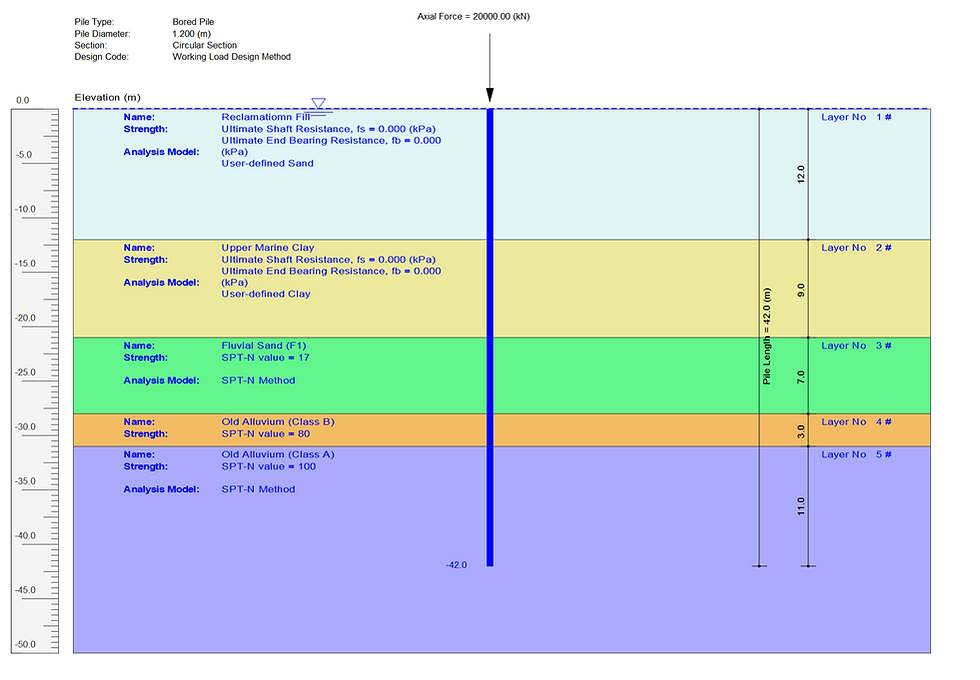top of page

# Bored pile socketed into interbedded clay and sand layers in Singapore

This blog presents a design example for a 1.2 m diameter reinforced concrete bored pile installed through interbedded clay and sand layers in Singapore. The typical subsurface profile at Marina Bay in Singapore consists of 12 m thick reclamation fill, 12 m thick very soft marine clay, 7 m thick fluvial sand followed by Class B old alluvium (very dense fine to medium grained silty sand) which overlies Class A old alluvium (very dense fine to coarse grained silty sand).

The figure below shows the ground profile with the pile length and loading conditions for this example. Axial force applied at the pile head is adopted to be 20 MN.The ground profile input dialog for this example is shown in the figure below. The groundwater table is adopted to be at the ground surface.The detailed soil layer input parameters for all the input soil layers are summarized in the following table. Both reclamation fill and upper marine clay are modelled with “user-defined sand” and “user-defined clay” models, respectively. The ultimate shaft resistance and ultimate end bearing resistance values for both layers are set as 0 to ensure that these two layers do not provide any contribution to the axial pile capacity.SPT-N method is used to model the other materials with the empirical factors to calculate the ultimate shaft resistance and ultimate end bearing resistance values. Note that the maximum shaft resistance is set to be 100 kPa for fluvial sand (F1) and 250 kPa for old alluvium materials based on the local experience. The maximum end bearing resistance is adopted to be 3000 kPa for fluvial sand (F1) and 7000 kPa for old alluvium materials.

Analysis option input parameters for this example are shown in the following figure. The pile type is selected to be “Bored Pile”. The maximum pile length is set as 42 m and the length increment number is adopted as 84. The axial loading for settlement analysis is adopted to be 20000 kN to calculate the load settlement curve. Working load design approach is adopted to be the pile capacity design code.The figure below shows the general output window of the PileAXL program for this example. Three axial capacity plot items and the tabulated results for the selected pile section are presented in the output window.The figure below shows the axial capacity plots for multiple pile sections with different section diameters. In this example, two other section diameters of 1.35 m and 1.5 m are adopted in the axial capacity calculation to demonstrate the useful features of the PileAXL program. The axial capacity results of different section sizes can be grouped into a single plot which helps the designers to select the reasonable pile size in the design.The figure below shows the spreadsheet calculation for the axial capacity based on the pile length of 42 m. The ultimate total shaft resistance is calculated to be 13526 kN. The ultimate bearing resistance for this pile length is 7917 kN. The total axial resistance is therefore calculated to be 21443 kN.The following table shows the comparisons of the analysis results calculated by the PileAXL program with the spreadsheet calculation.Four different runs using the PileAXL program are carried out with different pile element numbers. For the pile element number of 84, the pile element size adopted in the calculation is 0.5 m. The calculated total shaft resistance Qs (13290 kN) is about 98.3% of the estimation (13526 kN) from the spreadsheet calculation. Note that the difference becomes negligible with the increased pile element numbers. For Run 4, the difference between the PileAXL program and the spreadsheet calculation is less than 0.35% as shown in the table above.

167 views